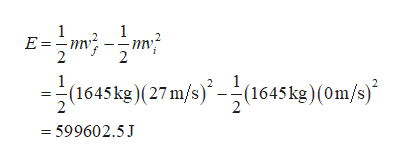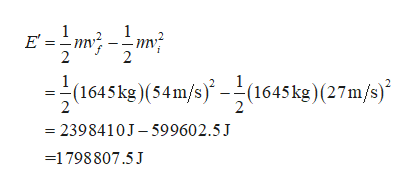What is the energy ?E required to accelerate a 1645 kg1645 kg car from rest to 27 m/s? Compared to the amount of energy required to accelerate a car from rest to 27 m/s,27 m/s, how much energy is required to accelerate the car from 27 m/s27 m/s to twice that speed, 54 m/s?

Question

What is the energy ?E required to accelerate a 1645 kg1645 kg car from rest to 27 m/s? Compared to the amount of energy required to accelerate a car from rest to 27 m/s,27 m/s, how much energy is required to accelerate the car from 27 m/s27 m/s to twice that speed, 54 m/s?

Step 1

From work energy theorem, the net work done is equal to change in kinetic energy .

Write the expression for energy required to accelerate 1645kg mass car from rest to 27m/s.help_outlineImage Transcriptionclose1 E m 1 (1645kg)(27 m/s)-(1645 kg) (0m/s) =599602.5J fullscreen
Step 2

Write the expression for energy required to accel...help_outlineImage Transcriptionclose1 E' = 2 2 (1645kg)(54m/s)-(1645kg)(27m/s) 2398410J-599602.5J 1798807.5J fullscreen

Want to see the full answer?

See Solution

Want to see this answer and more?

Our solutions are written by experts, many with advanced degrees, and available 24/7

See Solution
Tagged in

Physics